Скачать презентацию P E E R EARTHQUAKE SCIENCE-ENGINEERING INTERFACE STRUCTURAL

a701584ccf2dbc2a5fc94d926c0f6d03.ppt

• Количество слайдов: 25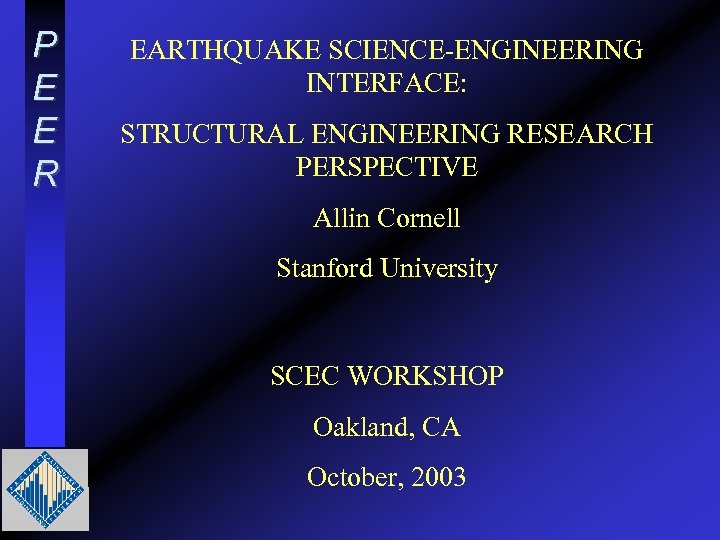P E E R EARTHQUAKE SCIENCE-ENGINEERING INTERFACE: STRUCTURAL ENGINEERING RESEARCH PERSPECTIVE Allin Cornell Stanford University SCEC WORKSHOP Oakland, CA October, 2003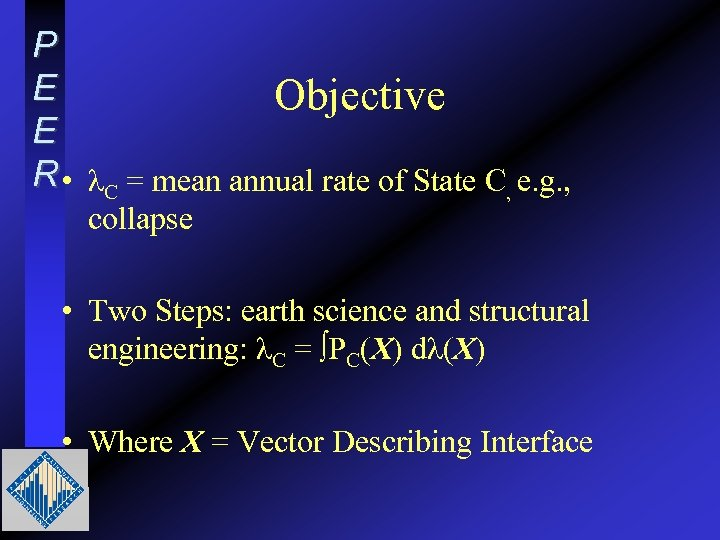P E Objective E R • λC = mean annual rate of State C, e. g. , collapse • Two Steps: earth science and structural engineering: λC = ∫PC(X) dλ(X) • Where X = Vector Describing Interface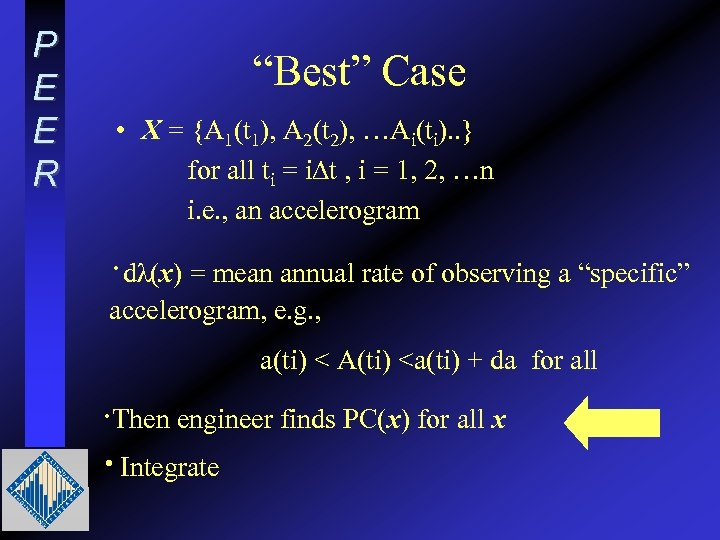P E E R “Best” Case • X = {A 1(t 1), A 2(t 2), …Ai(ti). . } for all ti = i∆t , i = 1, 2, …n i. e. , an accelerogram ·dλ(x) = mean annual rate of observing a “specific” accelerogram, e. g. , a(ti) < A(ti)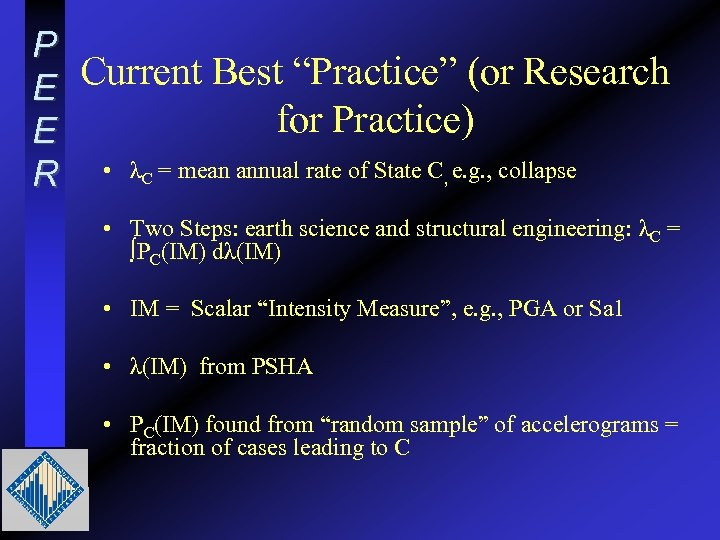P Current Best “Practice” (or Research E for Practice) E R • λC = mean annual rate of State C, e. g. , collapse • Two Steps: earth science and structural engineering: λC = ∫PC(IM) dλ(IM) • IM = Scalar “Intensity Measure”, e. g. , PGA or Sa 1 • λ(IM) from PSHA • PC(IM) found from “random sample” of accelerograms = fraction of cases leading to C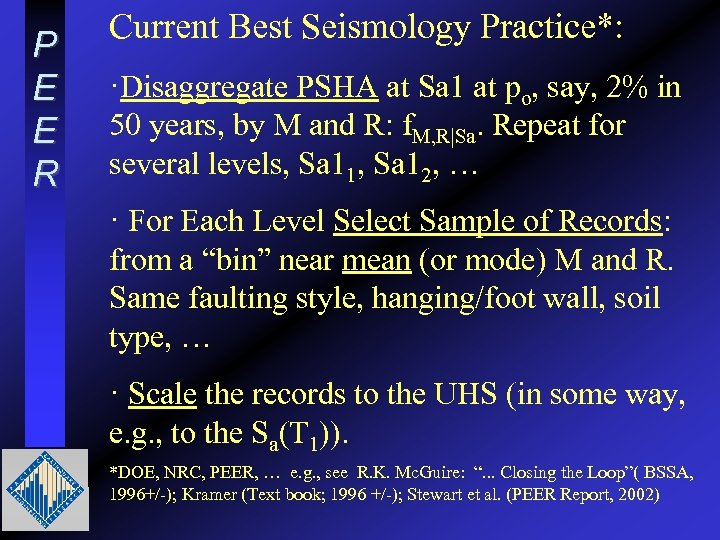P E E R Current Best Seismology Practice*: ·Disaggregate PSHA at Sa 1 at po, say, 2% in 50 years, by M and R: f. M, R|Sa. Repeat for several levels, Sa 11, Sa 12, … · For Each Level Select Sample of Records: from a “bin” near mean (or mode) M and R. Same faulting style, hanging/foot wall, soil type, … · Scale the records to the UHS (in some way, e. g. , to the Sa(T 1)). *DOE, NRC, PEER, … e. g. , see R. K. Mc. Guire: “. . . Closing the Loop”( BSSA, 1996+/-); Kramer (Text book; 1996 +/-); Stewart et al. (PEER Report, 2002)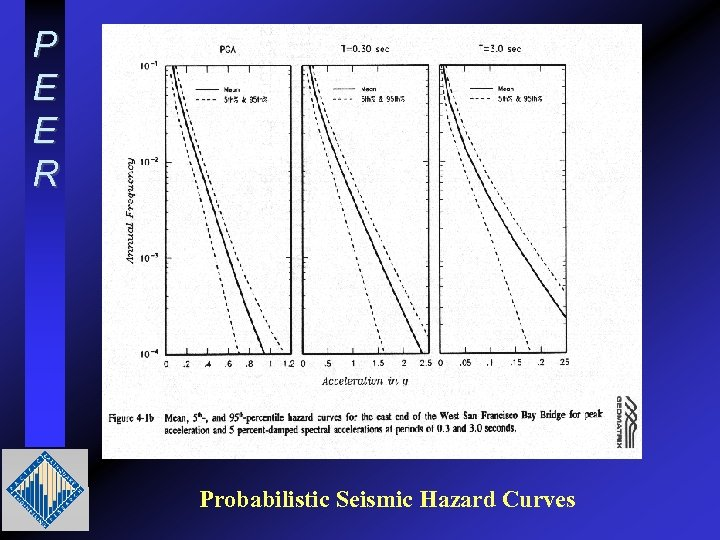P E E R Probabilistic Seismic Hazard Curves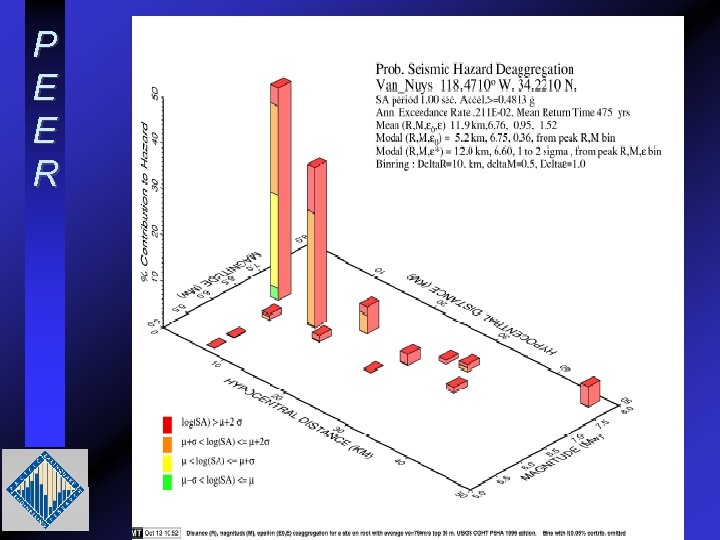P E E R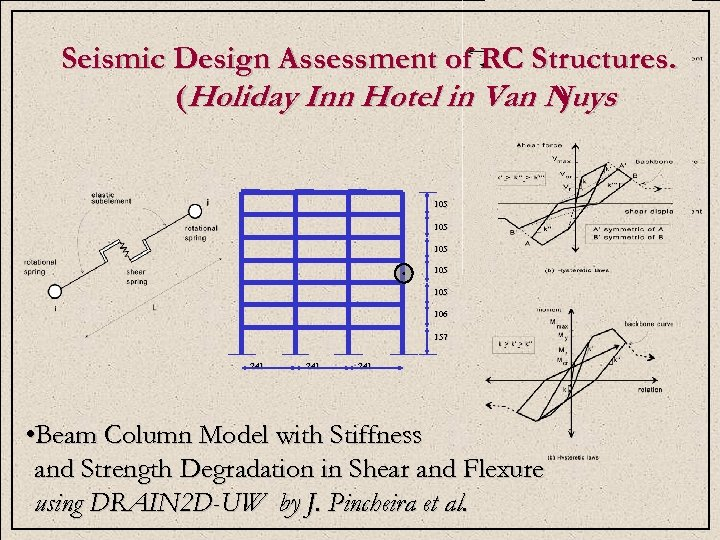P Seismic Design Assessment of RC Structures. E (Holiday Inn Hotel in Van Nuys ) E R 105 105 105 106 157 241 241 • Beam Column Model with Stiffness and Strength Degradation in Shear and Flexure using DRAIN 2 D-UW by J. Pincheira et al.P E E R Multiple Stripe Analysis C • The Statistical Parameters of the “Stripes” are Used to Estimate the Median and Dispersion as a Function of the Spectral Acceleration, Sa 1.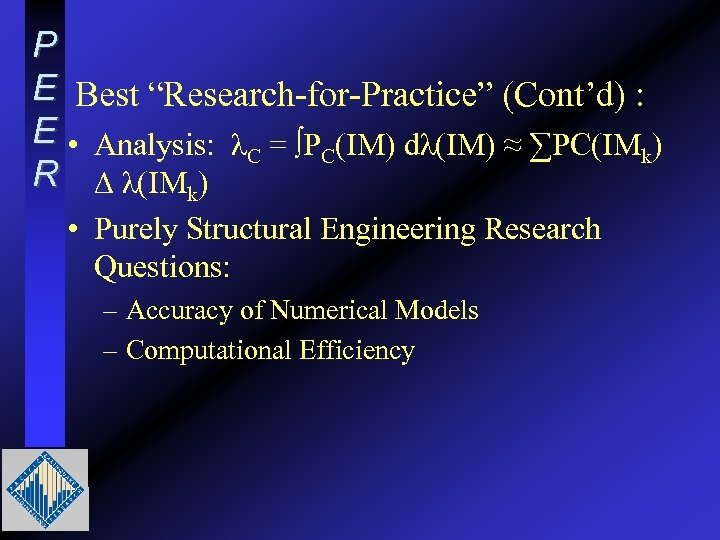P E Best “Research-for-Practice” (Cont’d) : E • Analysis: λ = ∫P (IM) dλ(IM) ≈ ∑PC(IM ) C C k R ∆ λ(IMk) • Purely Structural Engineering Research Questions: – Accuracy of Numerical Models – Computational Efficiency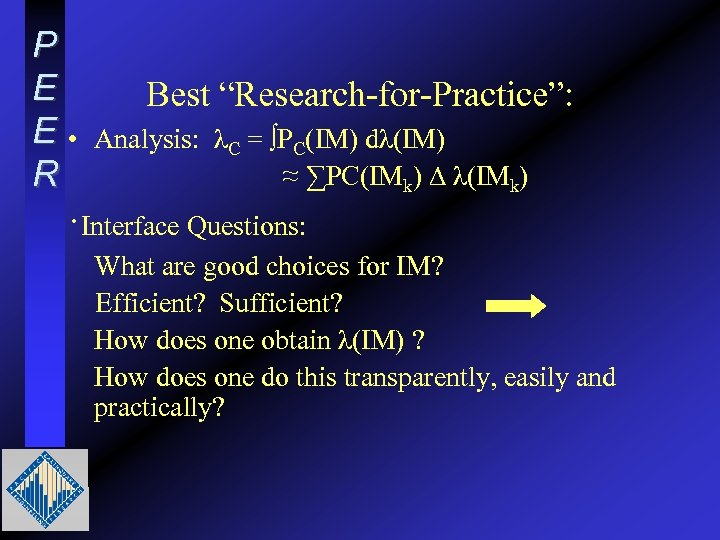P E E • R Best “Research-for-Practice”: Analysis: λC = ∫PC(IM) dλ(IM) ≈ ∑PC(IMk) ∆ λ(IMk) ·Interface Questions: What are good choices for IM? Efficient? Sufficient? How does one obtain λ(IM) ? How does one do this transparently, easily and practically?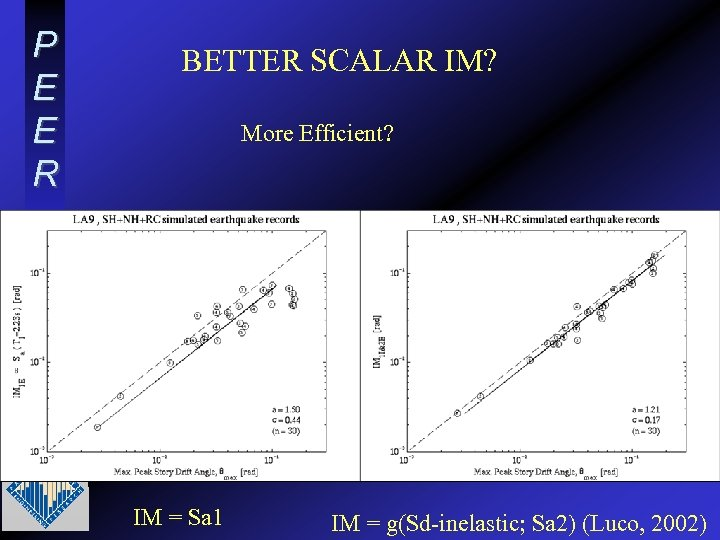P E E R BETTER SCALAR IM? More Efficient? IM = Sa 1 IM = g(Sd-inelastic; Sa 2) (Luco, 2002)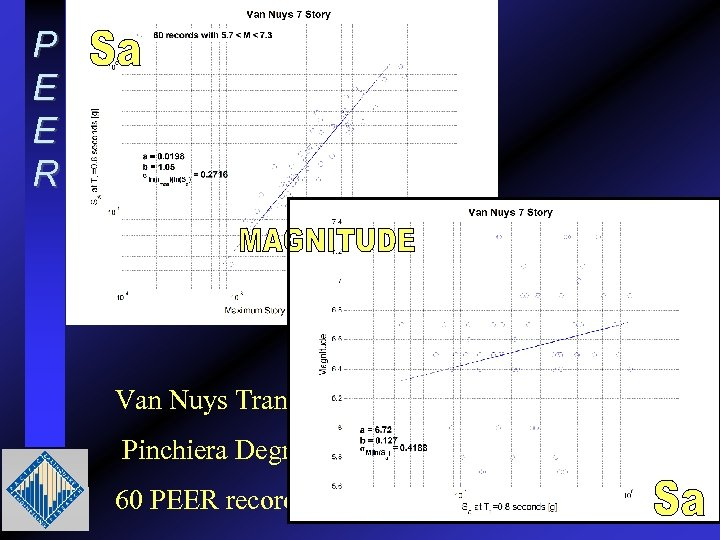P E E R Van Nuys Transverse Frame: Pinchiera Degrading Strength Model; T = 0. 8 sec. 60 PEER records as recorded 5. 3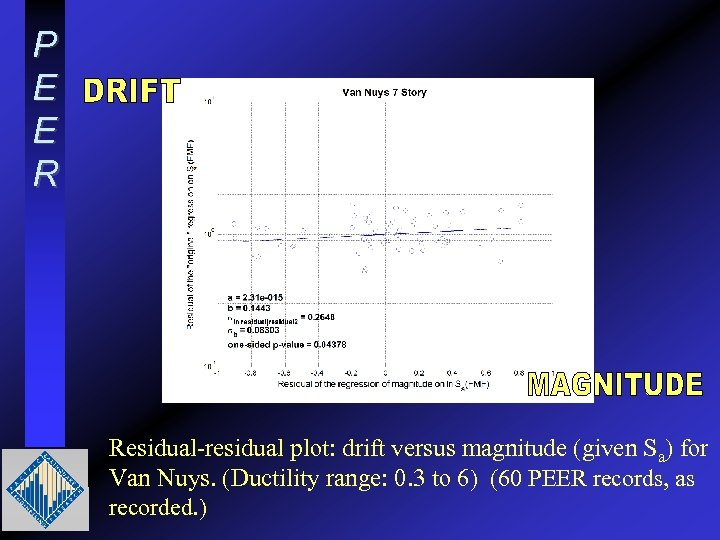P E E R Residual-residual plot: drift versus magnitude (given Sa) for Van Nuys. (Ductility range: 0. 3 to 6) (60 PEER records, as recorded. )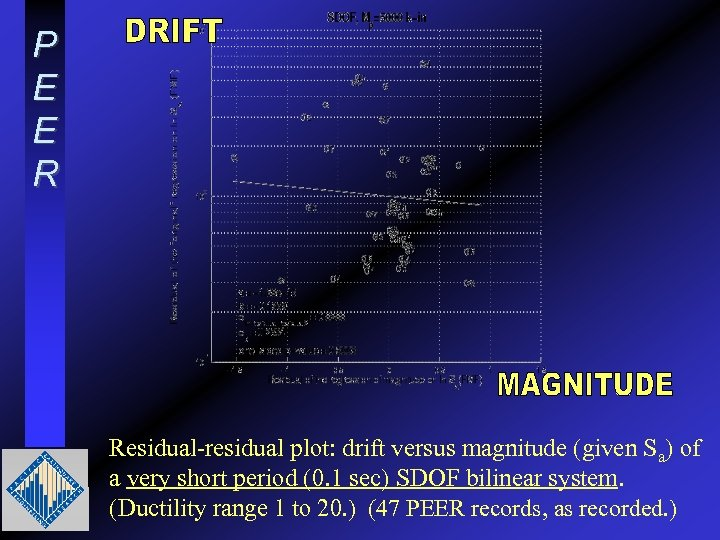P E E R Residual-residual plot: drift versus magnitude (given Sa) of a very short period (0. 1 sec) SDOF bilinear system. (Ductility range 1 to 20. ) (47 PEER records, as recorded. )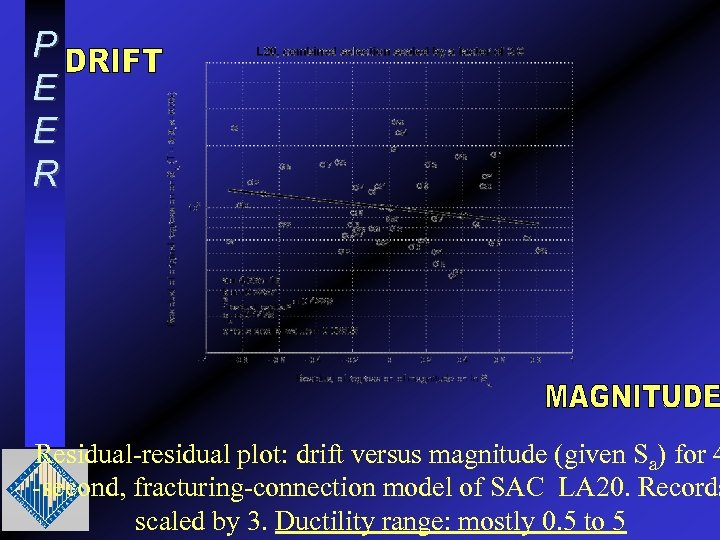P E E R Residual-residual plot: drift versus magnitude (given Sa) for 4 -second, fracturing-connection model of SAC LA 20. Records scaled by 3. Ductility range: mostly 0. 5 to 5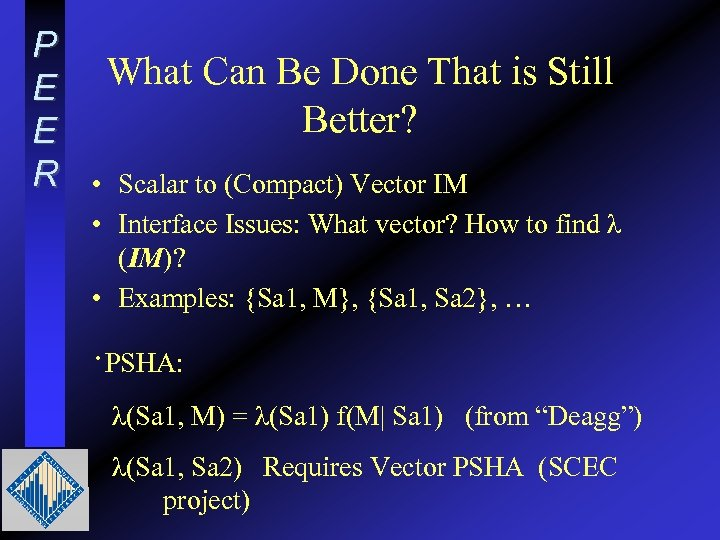P E E R What Can Be Done That is Still Better? • Scalar to (Compact) Vector IM • Interface Issues: What vector? How to find λ (IM)? • Examples: {Sa 1, M}, {Sa 1, Sa 2}, … ·PSHA: λ(Sa 1, M) = λ(Sa 1) f(M| Sa 1) (from “Deagg”) λ(Sa 1, Sa 2) Requires Vector PSHA (SCEC project)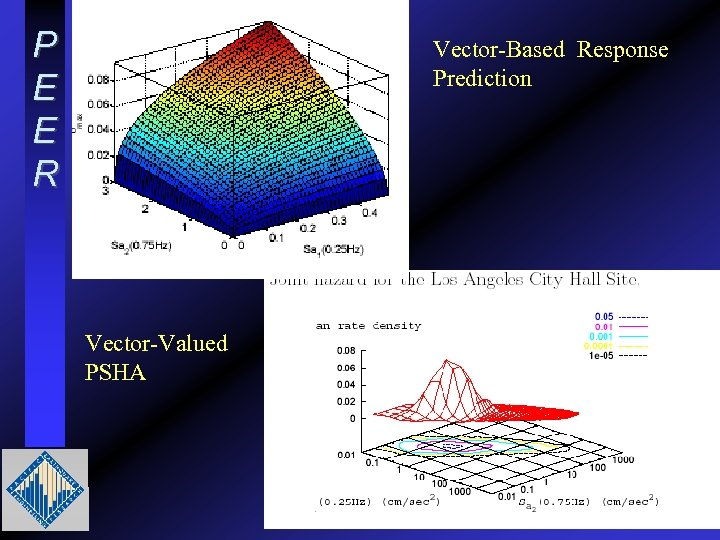P E E R Vector-Based Response Prediction Vector-Valued PSHA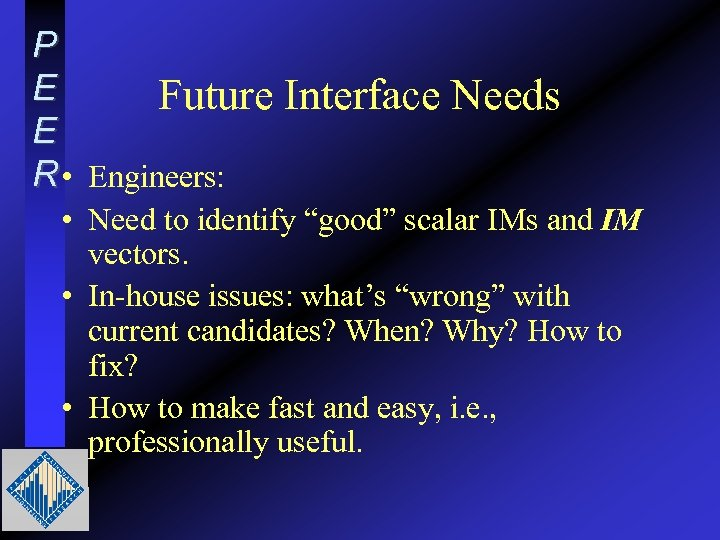P E Future E R • Engineers: Interface Needs • Need to identify “good” scalar IMs and IM vectors. • In-house issues: what’s “wrong” with current candidates? When? Why? How to fix? • How to make fast and easy, i. e. , professionally useful.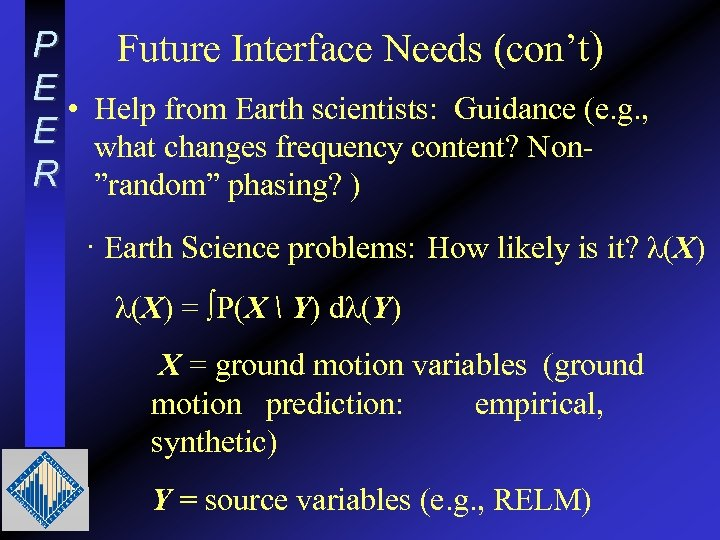P Future Interface Needs (con’t) E • Help from Earth scientists: Guidance (e. g. , E what changes frequency content? Non. R ”random” phasing? ) · Earth Science problems: How likely is it? λ(X) = ∫P(X Y) dλ(Y) X = ground motion variables (ground motion prediction: empirical, synthetic) Y = source variables (e. g. , RELM)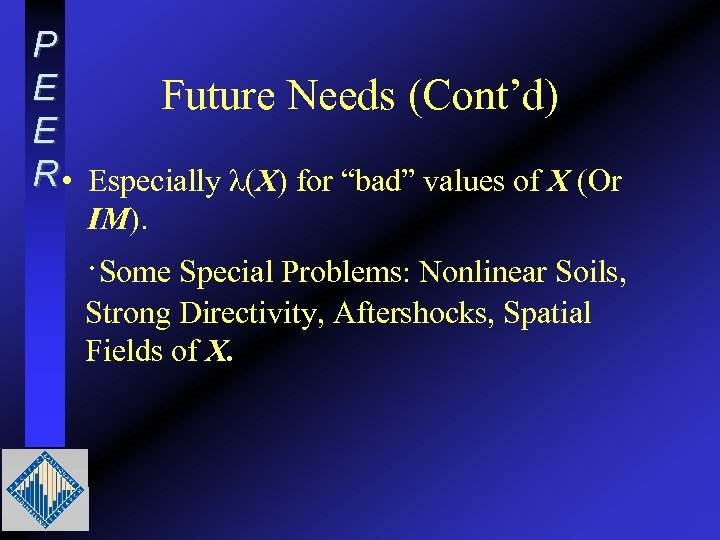P E Future Needs (Cont’d) E R • Especially λ(X) for “bad” values of X (Or IM). ·Some Special Problems: Nonlinear Soils, Strong Directivity, Aftershocks, Spatial Fields of X.P E E RP E E R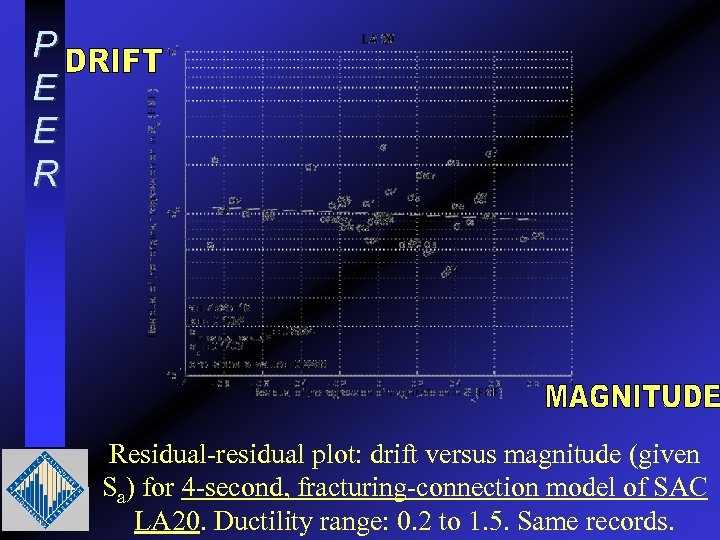P E E R Residual-residual plot: drift versus magnitude (given Sa) for 4 -second, fracturing-connection model of SAC LA 20. Ductility range: 0. 2 to 1. 5. Same records.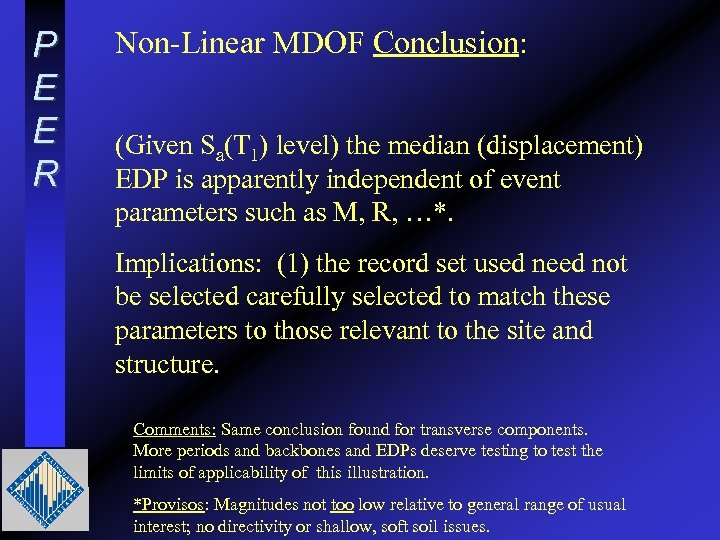P E E R Non-Linear MDOF Conclusion: (Given Sa(T 1) level) the median (displacement) EDP is apparently independent of event parameters such as M, R, …*. Implications: (1) the record set used need not be selected carefully selected to match these parameters to those relevant to the site and structure. Comments: Same conclusion found for transverse components. More periods and backbones and EDPs deserve testing to test the limits of applicability of this illustration. *Provisos: Magnitudes not too low relative to general range of usual interest; no directivity or shallow, soft soil issues.